Home > A2C > Chapter 13 > Lesson 13.2.2 > Problem13-118

13-118.
1. Prove that each of the following equations is an identity. In other words, prove that they are each true for all values for which the functions are defined. Homework Help ✎

1.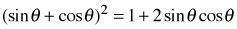2.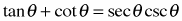3.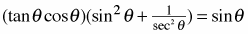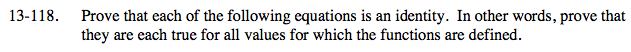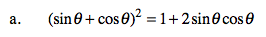sin2θ + cos2θ = 1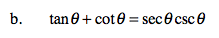Recall the trigonometric ratios for sinθ, cosθ, and tanθ.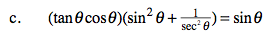Substitute trigonometric ratios for tanθ and sec2θ.
(sinθ)(sin2θ + cos2θ)
(sinθ)(1)
sinθ